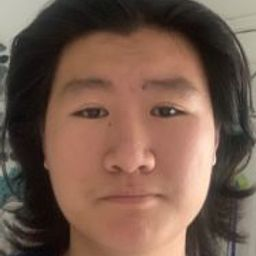# University Calculus: Early Transcendentals 4th

## Educators### Problem 1

Match the vector equations with the graphs (a)-(h) given here. (GRAPH CANT COPY)
$$\mathbf{r}(t)=t \mathbf{i}+(1-t) \mathbf{j}, \quad 0 \leq t \leq 1$$Jack H.

### Problem 2

Match the vector equations with the graphs (a)-(h) given here. (GRAPH CANT COPY)
$$\mathbf{r}(t)=\mathbf{i}+\mathbf{j}+t \mathbf{k}, \quad-1 \leq t \leq 1$$Jack H.

### Problem 3

Match the vector equations with the graphs (a)-(h) given here. (GRAPH CANT COPY)
$$\mathbf{r}(t)=(2 \cos t) \mathbf{1}+(2 \sin t) \mathbf{J}, \quad 0 \leq t \leq 2 \pi$$Jack H.

### Problem 4

Match the vector equations with the graphs (a)-(h) given here. (GRAPH CANT COPY)
$$\mathbf{r}(t)=t \mathbf{i}, \quad-1 \leq t \leq 1$$Jack H.

### Problem 5

Match the vector equations with the graphs (a)-(h) given here. (GRAPH CANT COPY)
$$\mathbf{r}(t)=t \mathbf{i}+t \mathbf{j}+t \mathbf{k}, \quad 0 \leq t \leq 2$$Jack H.

### Problem 6

Match the vector equations with the graphs (a)-(h) given here. (GRAPH CANT COPY)
$$\mathbf{r}(t)=t \mathbf{j}+(2-2 t) \mathbf{k}, \quad 0 \leq t \leq 1$$Jack H.

### Problem 7

Match the vector equations with the graphs (a)-(h) given here. (GRAPH CANT COPY)
$$\mathbf{r}(t)=\left(t^{2}-1\right) \mathbf{j}+2 t \mathbf{k}, \quad-1 \leq t \leq 1$$Jack H.

### Problem 8

Match the vector equations with the graphs (a)-(h) given here. (GRAPH CANT COPY)
$$\mathbf{r}(t)=(2 \cos t) \mathbf{i}+(2 \sin t) \mathbf{k}, \quad 0 \leq t \leq \pi$$Jack H.

### Problem 9

Evaluate $\int_{C}(x+y) d s,$ where $C$ is the straight-line segment $x=t, y=(1-t), z=0,$ from (0,1,0) to (1,0,0).Jack H.

### Problem 10

Evaluate $\int_{C}(x-y+z-2) d s,$ where $C$ is the straight-line segment $x=t, y=(1-t), z=1,$ from (0,1,1) to (1,0,1).Jack H.

### Problem 11

Evaluate $\int_{C}(x y+y+z) d s$ along the curve $\mathbf{r}(t)=2 t \mathbf{i}+$ $t \mathbf{j}+(2-2 t) \mathbf{k}, 0 \leq t \leq 1$.Jack H.

### Problem 12

Evaluate $\int_{C} \sqrt{x^{2}+y^{2}} d s$ along the curve $\mathbf{r}(t)=(4 \cos t) \mathbf{i}+$
$(4 \sin t) \mathbf{j}+3 t \mathbf{k},-2 \pi \leq t \leq 2 \pi$.Jack H.

### Problem 13

Find the line integral of $f(x, y, z)=x+y+z$ over the straightline segment from (1,2,3) to (0,-1,1).Jack H.

### Problem 14

Find the line integral of $f(x, y, z)=\sqrt{3} /\left(x^{2}+y^{2}+z^{2}\right)$ over the curve $\mathbf{r}(t)=t \mathbf{i}+t \mathbf{j}+t \mathbf{k}, 1 \leq t \leq \infty$.Jack H.

### Problem 15

Integrate $f(x, y, z)=x+\sqrt{y}-z^{2}$ over the path $C_{1}$ followed by $C_{2}$ from (0,0,0) to (1,1,1) (see accompanying figure) given by
$$\begin{array}{ll}C_{1}: & \mathbf{r}(t)=t \mathbf{i}+t^{2} \mathbf{j}, \quad 0 \leq t \leq 1 \\C_{2}: & \mathbf{r}(t)=\mathbf{i}+\mathbf{j}+t \mathbf{k}, \quad 0 \leq t \leq 1\end{array}$$
(GRAPH CANT COPY)
The paths of integration for Exercises 15 and 16Jack H.
Integrate $f(x, y, z)=x+\sqrt{y}-z^{2}$ over the path $C_{1}$ followed by $C_{2}$ followed by $C_{3}$ from (0,0,0) to (1,1,1) (see accompanying figure) given by